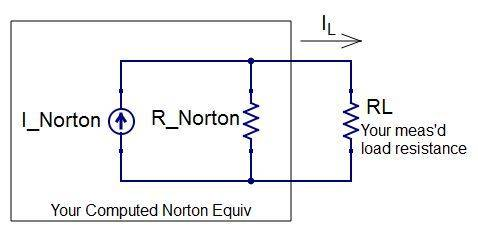# Current Division

Marcin H

## Homework StatementFind the load resistor current for 4 different resistors.

Current Division

## The Attempt at a Solution

I need to use current division to find the load resistor current for 4 different resistors, but I am not sure how to apply it here. I have I_norton and R_norton and the RL is also given as one of the resistors (560ohms for example). How can I apply current division here?

axmls
What about the current division is giving you issues? Have you applied the current division equation before? What are the important quantities you need to look at to apply current division?

Marcin H
What about the current division is giving you issues? Have you applied the current division equation before? What are the important quantities you need to look at to apply current division?
I haven't had much experience with it. Looking off an example from my notes, I think this would be it.

Rx being the 560ohm resistor for example. Is that right?

EDIT: changed Rth to RN*******

axmls
I'm assuming Rth is your Norton resistor?

This isn't exactly correct, though the form is correct. To see why, let's assume you have the same circuit you have right now, but assume your load resistor is HUGE, and your norton equivalent resistance is small. Then qualitatively, you could see that most of the current would go through the Norton resistor (it's a path of less resistance). Then let's apply your current division equation to this situation to get the current through the load:
$$I_L = I_N \frac{R_L}{R_N + R_L}$$
But notice that this fraction is very close to ##1## (because the load resistor is huge and the Norton resistance is small), so this equation tells us that most of the current from the current source goes through the load, but obviously this can't be correct if the load resistance is much higher than the Norton resistance.

So, you'll have to make a modification to your current division equation.

Marcin H
So, you'll have to make a modification to your current division equation.

IL = (I_Norton*R_Norton/(RL + R_Norton))

Is that correct?

axmls
Correct. Just remember that with current division, the current is multiplied by the resistance of the branch whose current you don't want divided by the sum of the two branch resistances. Looking at extreme cases like I did above can help you to remember.

•Marcin H